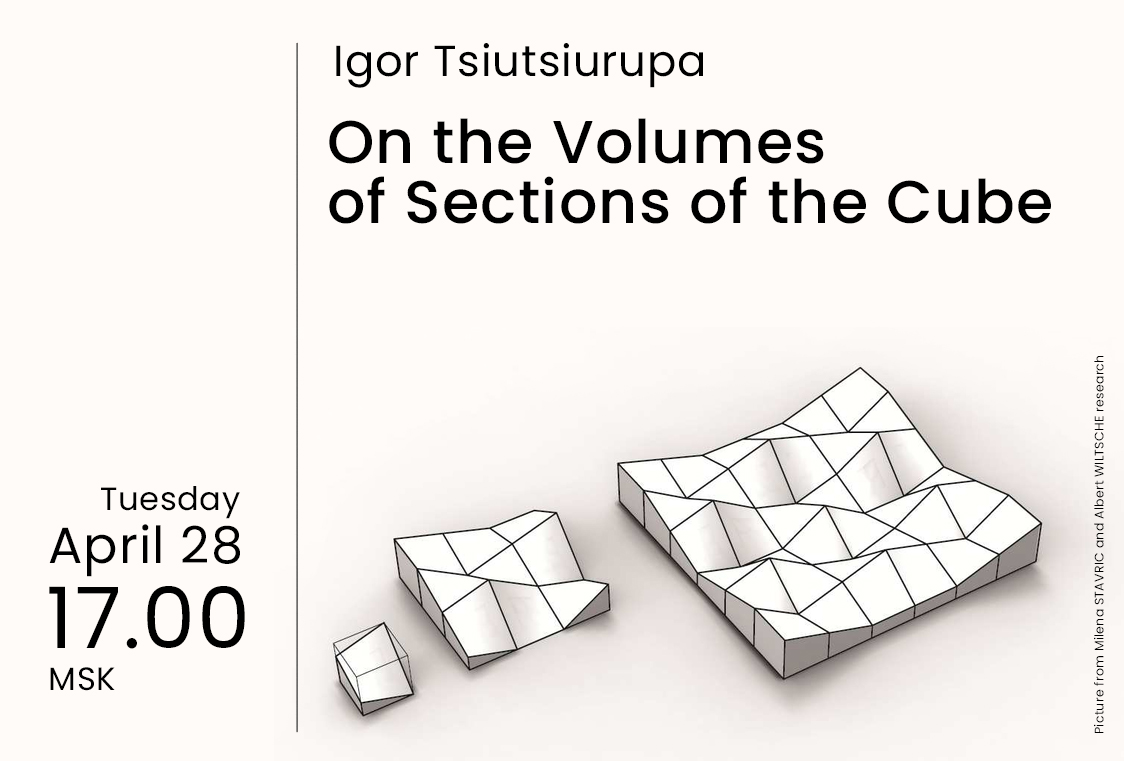# Igor Tsiutsiurupa “On the Volumes of Sections of the Cube”April 28, 2020
17.00 - 18.30 MSK (UTC +3)

# Igor Tsiutsiurupa "On the Volumes of Sections of the Cube"

This tuesday Igor Tsiutsiurupa will give the talk "On the Volumes of Sections of the Cube" based on the joint work with G. Ivanov.

The talk will be held in zoom
Meeting ID: 897-2880-0784
Password: first 6 decimal places of $\pi$

Abstract:

It is widely known that a k-dimensional convex centrally symmetric polytope is affine equivalent to a central section of a higher dimensional cube. It is convenient to consider the sections of the cube as a classical position of the convex centrally symmetric polytopes. Therefore, the problems related to the volume of such polytopes are connected with the volume of the sections of the cube.

The classical result is the Vaaler theorem (1997), which says that the minimal volume of a k-dimensional section of the n-cube is that of k-cube. In 1986, Ball obtained the upper bound on the volume of a k-dimensional section which is tight whenever k divides n or n is at most 2k.

We show how the problem of finding the k-dimensional section of the maximal volume can be stated in linear algebraic terms that reduce the problem to perturbations of polytopes.

We will discuss simple algebraic conditions that rise as part of this approach. We find the maximal volume of a planar section of the n-cube for n>2.

## Watch the video:

Everyone is invited to attend. The language of the lecture is English. The event is aimed at master and graduate students, as well as researchers in the field of combinatorics.